# Primary Storage

View Only

### First Step Into Quantum Computing: Hello World

#### By Archive User posted Fri November 01, 2019 01:11 PM

Originally posted by: Th. Mühge

The ‘paradox’ is only a conflict between reality and your feeling of what reality ‘ought to be’“
Richard Feynman

I was on the IBM Systems Technical University 2019 in Prague, were I got the chance to try out programming IBM Q for the first time.  It is amazing, that it is already possible to login into such a machine from a conference room and start manipulating quantum bits also called qubits.

OK, being a Physicist makes it even more exciting for me, although over time my knowledge in Quantum Mechanics almost disappeared. Honestly, it seems to be essential to deep dive into this quantum world before being able to write meaningful programs.

Anyway forget about all these obstacles and start!

I logged into the IBM Qiskit open-source quantum computing software development framework website.

https://qiskit.org/

I setup my account and was able to start!
Now I have the possibility to begin the quantum journey via a notebook or via a Graphical User Interface (GUI).
To start, I will go for the GUI, to make things easier.

So, what do I have now?
I have 5 quantum bits or qubits in front of me (q,…q), that I can manipulate. All of them are initialized to the state [0>. I also have a whole lot of quantum gates and operations that I can use to write programs.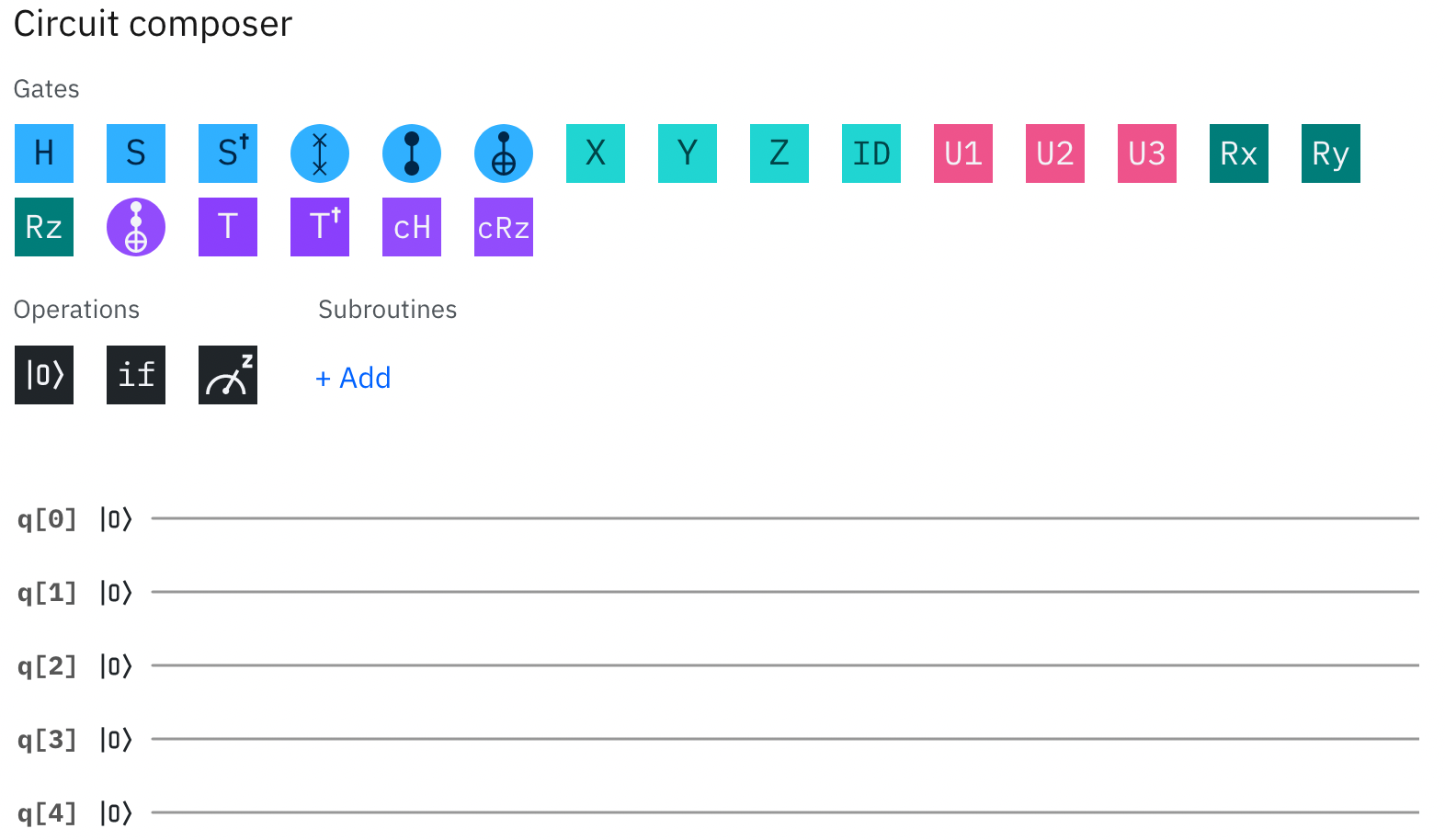How to understand the states and measurements in the quantum world:
Classically one performs a measurement to determine the state.
Example: A coin has two states being a head and a tail. The coin can only have these two states. Nothing in-between. A measurement will show one of the two states.
The equivalent of this two state system would be a classical bit with the two possible states 0 and 1.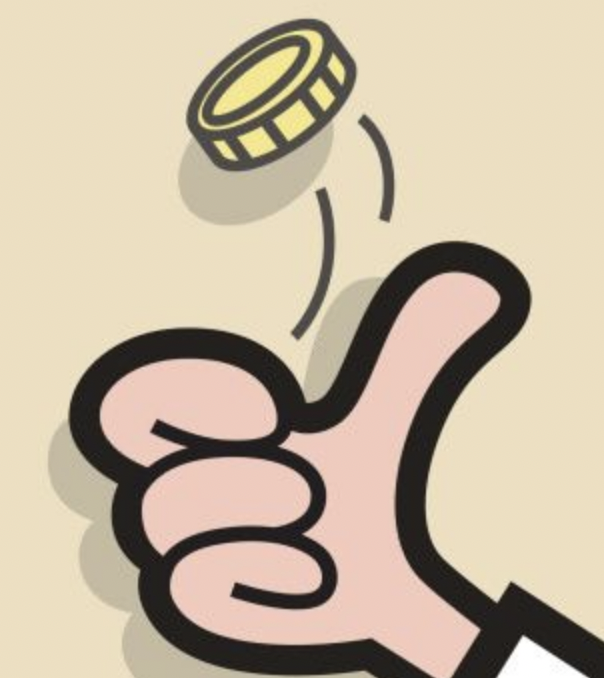Going into the quantum world this is not true any more. States and measurements are different and their relationship is not intuitive any more.

Excursion:
Lets recall complex numbers. Some people might remember how useful it is in electronic engineering to work with complex numbers when dealing with
alternated current (AC)  circuits. Now in contrast to direct current (DC), physical parameters like e.g. voltage and current become dynamic and in order to describe them the frequency and phase come into play in addition.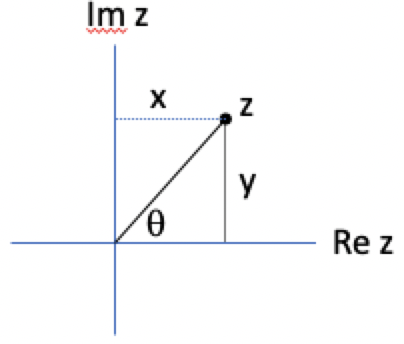Voltage and current are alway real observable parameters (Re z only). However introducing the imaginary part (Im z) makes AC circuit analysis a lot less complex.

Going back to the quantum world and qubits:
Different from a classical bit the qubit can rotate in space in the x,y and z direction.

The below picture is a geometrical representation of our single qubit, called the Bloch Sphere (drawn in quikit by: "plot_bloch_vector([1, 1, 1]").
Now in principal instead of being in the state 0 or 1 like a classical bit the qubit can have infinite states on this Bloch sphere.
The strange thing is now however, that a measurement causes the qubit state always to collapse into either a 0 or a 1.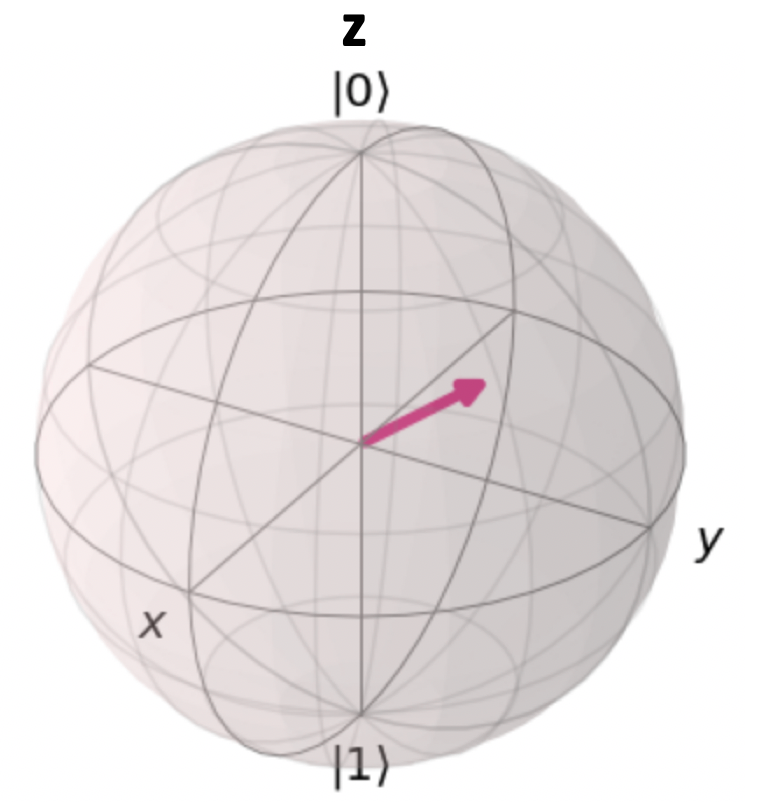Now we have the qubit and and we need some machine to perform a measurement (our machine will be cat free :-) )
With this machine we need to decide the direction of our measurement e.g. the x,y or z direction.
Let’s choose the z direction and start a measurement.
Our measurement will show either a 0 or a 1 as stated before. Lets assume a 1 means that our qubit points upwards along the z axis and that a 0 means that our qubit points downwards.
If we subsequently repeat the measurement the previous result will be repeated.

At this point everything is not too exciting, but what happens if we are able to rotate our qubit by 90 degrees from the z into the x-y direction and subsequently measure its state along the z-axis?
Our qubit can only have a state 0 or a state 1.
Now we are at a point were our classical interpretation is not valid any more.

Lets stop the discussion and let us go back to our quantum computer…..

As a quantum „Hello World“ experience we will perform the above outline experiment with our qubit q.
Qubit q is already aligned along the z direction with a state 0.
I will perform the 90 deg rotation away from the z axis of our qubit q by using the Hadamard (H) gate.
Afterwards I will perform a measurement in z-direction to see the outcome of our first „Hello World“ experiment.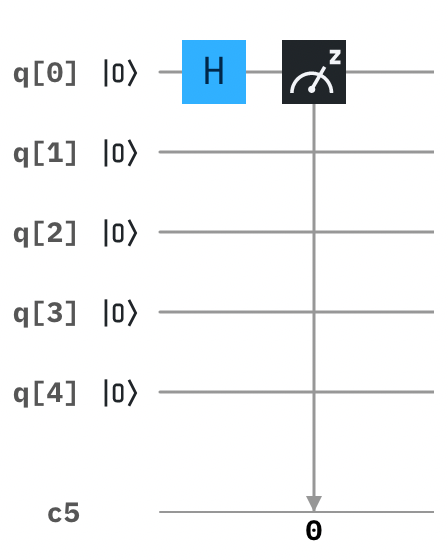I save my simple circuit and press the GUI run button.
Now I can decide to run the little experiment on a Quantum simulator, or on a real IBM Quantum system. I also need to decide on the number of repetitions.
To start with I go for the simulator and for 1024 repetitions.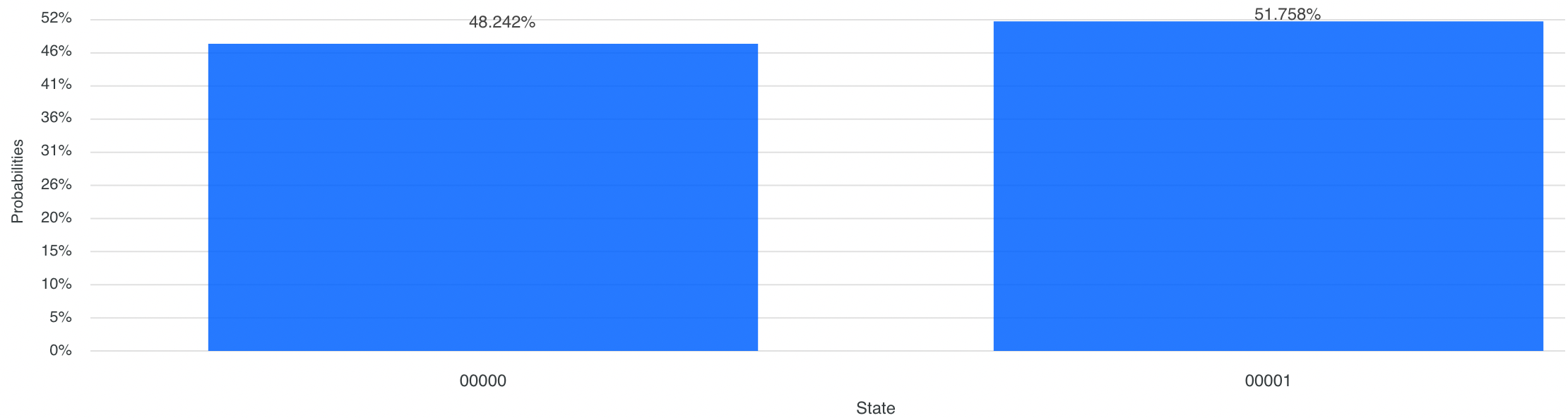How to I interpret the outcome?
I see that a state 0  and a state 1 are measured with almost a 50% probability each. The outcome of a measurement can only be a state 0 or a state 1.
Our qubit q is pointing with 50 % probability upwards (state 0) and with 50 % probability downwards (state 1).
The average (50% up - 50 % down) is zero which equals to the projection of q towards the z-axis.

Why we do not see exactly a 50% shared probability?
This is due to the noise within the quantum computer, that was also introduced to the simulator I used in the present case.

What if I wanted to rotate our qubit q by e.g. 45 degrees?
We will perform this experiment using a Rotation or R gate.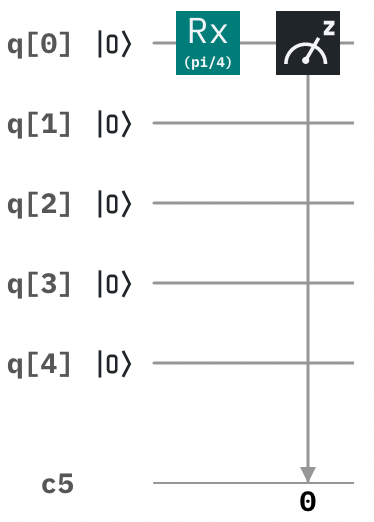Again I save the experiment and run it with 1024 repetitions.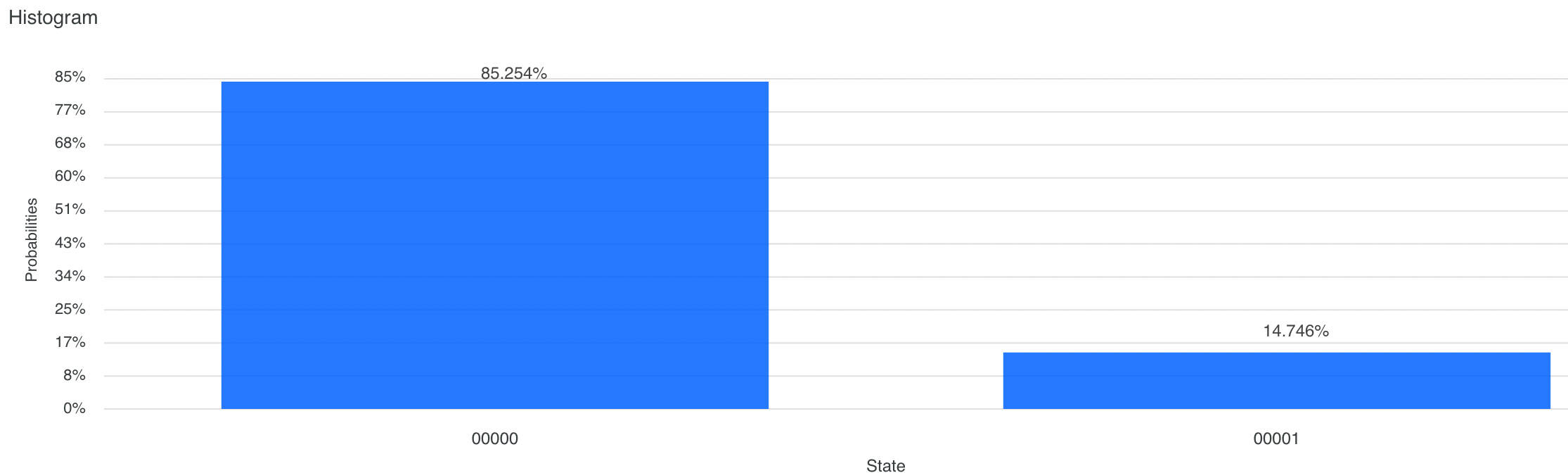How do I interpret this result having a 85 % probability for a 0 (up) and a 15 % probability for a 1 (down)?
Well, we performed a rotation of 45 degrees away from z.
From trigonometry we know that the projection of our qubit towards the z-axis would be the sin(45deg) which equals to 0.7.
The average of the up and down results of this experiment is 85% - 15 % which equals to 0.7 so we can derive qubit rotation from probabilities of our measurement outcome.

As a summary we could derive from our „Hello World“ experience that qubits are not deterministic.
A single experiment can be statiscally random.
Multiple repetitions of experiments are necessary and the averages of this results somehow point towards something we know from our all day world.

References:

https://community.qiskit.org/textbook/

Leonard Susskind & Art Friedman: "Quantum Mechanics the Theoretical Minimum"; Penguin Science.